##### Algebra II For Dummies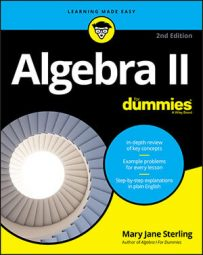Any equation with one or more rational terms (or fractions) is a rational equation. Although these equations aren't always easy to handle, you can turn them into quadratic equations by finding their least common denominator, or LCD.

To solve a rational equation with the LCD, you find a common denominator, write each fraction with that common denominator, and then multiply each side of the equation by that same denominator to get a nice quadratic equation.

Quadratic equations can have two solutions, so they present more opportunities for extraneous solutions. Be on the lookout!

By breaking down this approach into the following steps you can solve scary-looking rational equations like this one: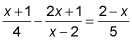1. Find a common denominator for all the terms in the equation.

In this example, the common denominator of all three fractions in the equation consists of the product of all the factors in the three denominators, 20(x – 2).

Each of the denominators has to be able to divide into the common denominator evenly. In other words, the LCD is a multiple of each of the original denominators. To solve this equation, 20(x – 2) is the common denominator, because it's a multiple of 4 — you multiply by 5(x – 2) to get it; it's a multiple of (x – 2) — you multiply by 20 to get it; and it's a multiple of 5 — you multiply by 4(x – 2) to get it. All three denominators divide this product evenly.

2. Write each fraction with the common denominator.

Multiply each of the terms in the original equation by some value so that, after the multiplication, each resulting term has the same denominator — the LCD you're so fond of: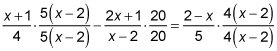The "some value" is equal to one, because each of the fractions multiplying the terms is the same in the numerator and denominator.

Now, multiplying each fraction and simplifying,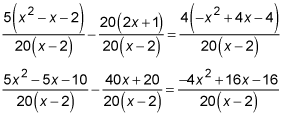3. Multiply each side of the equation by that same denominator.

This reduces each term and gets rid of the denominators: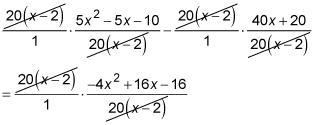Now simplify what's left.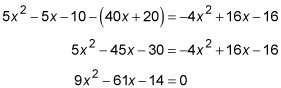4. Solve the new equation.

By completing the previous steps, you have produced a quadratic equation. To solve it, you can either factor or use the quadratic formula. This equation factors into (9x + 2)(x – 7) = 0.

After factoring, you set each factor equal to zero and solve for x. When 9x + 2 = 0,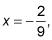and when x – 7 = 0, x = 7.

Check to be sure that both your solutions work in the original equation; one or both may be extraneous solutions.

The most common indication that you have an extraneous solution is that you end up with a zero in the denominator after replacing all the variables with that answer. Occasionally, you get a "nonsense" equation such as 4 = 7 when checking — and that tells you that the solution is extraneous — but those are very special cases. You should always check your answers after solving equations. Make sure that the value(s) you find create true statements.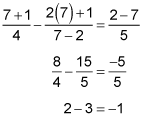When replacing the x in the original equation with the two solutions, they both work.